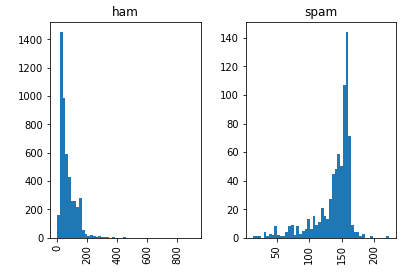15 Jul, 2019 Root User

In this tutorials, we are going to implement spam detection on message with given data sets. The dataset is in the csv format and it can be downloaded from github links

import python pandas library to read and manipulate data

``````
import pandas as pd
```
```

The downloaded dataset is placed in project-path/csv/EnglishSpam.csv

``````
filename=r'csv/EnglishSpam.csv'
```
```

Read the csv file using pandas. Here we are using the separator as `\t` as because the columns in pandas dataframe is separated by space. Usually the columns are separated by `;` or ':'.

``````
data = pd.read_csv(filename,sep="\t",header=None)
```
```

We label the columns name as label and message as because there was no name.

``````
data=data.rename(columns = {0:'label',1:'message'})
# print(data.head())
```
```

This is the important part. We have to explore the features so that we can distinguish between spam and non-spam 'ham' messages. Here we are interested in length of messages, because It could be a feature that affect the spam and non-spam in this datasets.

``````
data['length'] = data['message'].map(lambda text: len(text))
```
```

Let's plot the histogram of length

``````
data.hist(column='length', by='label', bins=50)
``````

Let's split the data into training and testing sets

``````
from sklearn.model_selection import train_test_split
labels=data["label"]
features=data[["length"]]
train, test, train_labels, test_labels = train_test_split(features,labels,test_size = 0.40, random_state = 42)
```
```

Build the model using bayes

# Build the model # Gaussian
``````
from sklearn.naive_bayes import GaussianNB
gnb = GaussianNB()
model = gnb.fit(train, train_labels)
```
```

Measure the accuracy

``````
preds = gnb.predict(test)
print(preds)
```
```

Text Accuracy

``````
from sklearn.metrics import accuracy_score
print(accuracy_score(test_labels,preds))
``````Prosperous Nepal is possible only from technological innovation.

© 2021, All right reserved.